Download Presentation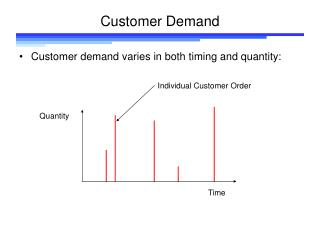Customer Demand

# Customer Demand - PowerPoint PPT Presentation

Customer Demand. Customer demand varies in both timing and quantity:. Individual Customer Order. Quantity. Time. Customer Demand.I am the owner, or an agent authorized to act on behalf of the owner, of the copyrighted work described.
Download Presentation## Customer Demand

An Image/Link below is provided (as is) to download presentation

Download Policy: Content on the Website is provided to you AS IS for your information and personal use and may not be sold / licensed / shared on other websites without getting consent from its author.While downloading, if for some reason you are not able to download a presentation, the publisher may have deleted the file from their server.

- - - - - - - - - - - - - - - - - - - - - - - - - - E N D - - - - - - - - - - - - - - - - - - - - - - - - - -
Presentation Transcript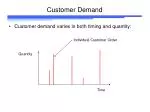Customer Demand
• Customer demand varies in both timing and quantity:

Individual Customer Order

Quantity

Time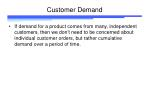Customer Demand
• If demand for a product comes from many, independent customers, then we don’t need to be concerned about individual customer orders, but rather cumulative demand over a period of time.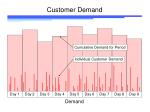Customer Demand

Cumulative Demand for Period

Individual Customer Demand

Day 3

Day 4

Day 5

Day 6

Day 7

Day 8

Day 9

Day 1

Day 2

Demand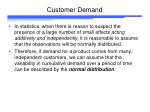Customer Demand
• In statistics, when there is reason to suspect the presence of a large number of small effects acting additively and independently, it is reasonable to assume that the observations will be normally distributed.
• Therefore, if demand for a product comes from many, independent customers, we can assume that the variability in cumulative demand over a period of time can be described by the normal distribution.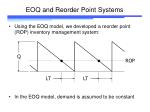Q

ROP

LT

LT

EOQ and Reorder Point Systems
• Using the EOQ model, we developed a reorder point (ROP) inventory management system:
• In the EOQ model, demand is assumed to be constant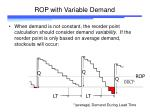Q

Q

Q

ROP with Variable Demand
• When demand is not constant, the reorder point calculation should consider demand variability. If the reorder point is only based on average demand, stockouts will occur:

ROP

DDLT*

LT

LT

*(average) Demand During Lead Time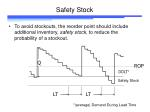Q

Safety Stock
• To avoid stockouts, the reorder point should include additional inventory, safety stock, to reduce the probability of a stockout.

ROP

DDLT*

Safety Stock

LT

LT

*(average) Demand During Lead Time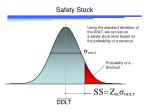DDLT

Safety Stock

Using the standard deviation of

the DDLT, we can set an

a safety stock level based on

the probability of a stockout

Probability of a

Stockout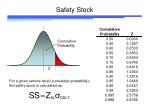Cumulative

Probability

Z

Safety Stock

For a given service level (cumulative probability),

the safety stock is calculated as: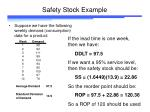If the lead time is one week,

then we have:

If we want a 95% service level,

then the safety stock should be:

So the reorder point should be:

So a ROP of 120 should be used

DDLT = 97.5

SS = (1.6449)(13.9) = 22.86

ROP = 97.5 + 22.86 = 120.36

Safety Stock Example
• Suppose we have the following weekly demand (consumption) data for a product:Safety Stock using MAD
• Many times, Safety Stock levels are calculated using the Mean Absolute Deviation as a measure of variability rather than the Standard Deviation. There are two reasons for this:
• Historical: Before calculators, the calculation of a standard deviation was not a trivial task, while the calculation of the Mean Absolute Deviation is fairly simple to perform by hand
• Robustness: The Mean Absolute Deviation measure is not as easily affected by outlier points as it is using the absolute value of the deviation rather than the squared deviationWeek DemandSafety Stock using MAD
• The standard deviation can be estimated from the MAD using:
• As a result, we can define a safety factor R which can be used to determine the safety stock based on the MAD and the desired service level:

SD = 1.25 MADSafety Stock Example Revisited
• The following weekly demand (consumption) data for the product was:

The Demand During Lead Time is:

For a 95% service level,

the safety stock should be:

So the reorder point should be:

So a ROP of 119 should be used

(vs. 120 calculated using the SD)

DDLT = 97.5

SS = (2.0561)(10.4) = 21.38

ROP = 97.5 + 21.38 = 118.88Demand Period vs. Lead Time Period
• In the previous example, the demand period (the period of time used to accumulate customer demand) was one week, which was the same as the lead time.
• Suppose the lead time was two weeks. Then the variability of the demand for a two week period would be greater than the MAD calculated from demand data aggregated weekly.
• We have assumed that customer demand is independent, i.e. that the demand for the product comes from a number of unrelated customers. In that case, then we can use a theorem from statistics to determine the appropriate variability of demand during lead time when the demand period is different from the lead time periodDemand Period vs. Lead Time Period
• Suppose we have two independent, normally distributed random variables:
• X: mean X, standard deviation X
• Y: mean Y, standard deviation Y
• Then the sum of these variables, Z = X + Y has mean:
• Z = X + Y

and standard deviationDP = 1 week

LT = 2 weeks

Demand Period vs. Lead Time Period
• Suppose that the demand period is 1 week (customer demand is measured on a weekly basis) and the lead time is two weeks. Then the standard deviation for the lead time can be calculated as:Demand Period vs. Lead Time Period
• Suppose that the demand period is 2 weeks (customer demand is accumulated in 2 week intervals) and the lead time is one week. Then the standard deviation for the lead time can be calculated as:

DP = 2 weeks

LT = 1 weeksSafety Stock

In general terms, the standard deviation of the demand

for the lead time is:

where the lead time and demand period are measured in

the same time units (typically days). The demand period is

level of aggregation used for determining demand.Safety Stock

So the safety stock level can be calculated as:

using the standard deviation of demand and:

using the MAD, where:DDLT

Note that if the Demand Period does not equal the Lead

Time, then the DDLT is calculated as:Safety Stock: Example 1

Demand data for a material has been collected on a weekly

basis for 6 months. Demand appears level, with:

Mean: 270 units/week

Standard deviation: 40 units/week

The lead time is 10 days. Calculate the safety stock

required for a 99% customer service level.Safety Stock: Example 1
• The formula for safety stock using the standard deviation is:

so for this example we have:Safety Stock: Example 2

Demand data for product has been collected on a weekly

basis with the following results:

Mean: 109 units/week

The lead time is 4 days. Calculate the safety stock

required for a 95% customer service level.Safety Stock: Example 2
• The formula for safety stock using the standard deviation is:

so for this example we have:Demand Period and Lead Time in SAP

Demand period is set by the Period Indicator on the Forecasting View

of the Material Master

The applicable periods are:

M – Monthly

W – Weekly

T – DailyDemand Period and Lead Time in SAP

In-house production is used for

Plnd delivery time + GR processing time +

Purchasing proc. time is used for

Lead Time for externally procured materialsLT

LT

LT

Exposure to Stockout
• Stockouts usually occur when stock gets low—for example, during the lead time period before a new order arrives:

Periods of maximum exposure to stockoutLT

LT

LT

LT

LT

LT

LT

LT

LT

LT

Exposure to Stockout
• The more frequently we order, the more chances there are of stocking out.

Twice as many

opportunities for

stockoutExposure to Stockout
• To fully evaluate the customer service level, we should calculate the customer service level on an annual basis:

where D is annual demand and Q is the order quantity.Exposure to Stockout
• For example, if we used a service level of 95% in calculating the safety stock, the annual demand D is 12,000 units and the order quantity Q is 800 units, then we have:
• So there is only a 46.3% chance of going a year without a stockoutRegular Demand

Day 3

Day 4

Day 5

Day 6

Day 7

Day 8

Day 9

Day 1

Day 2

Week 2

Week 1

Day 3

Day 4

Day 5

Day 6

Day 7

Day 8

Day 9

Day 1

Day 2

Week 1

Week 2

Sparse Demand

Demand PatternsDemand Patterns
• In developing the safety stock calculations, it was assumed that demand was generated from a “large” number of independent sources, and
• The individual demands are aggregated over a time period sufficiently long so that there are a number of individual demands contributing to each period demand.
• If these conditions are not met, then the safety stock values may not perform as expected.Demand Patterns
• If demand is sparse, then a more detailed approach to inventory planning that considers the expected time between orders as well as the expected order quantity

Quantity

Expected

order

quantity

Expected time

between orders

Time